# Maharashtra Board 11th Commerce Maths Solutions Chapter 5 Correlation Ex 5.1

Balbharati Maharashtra State Board 11th Commerce Maths Solution Book Pdf Chapter 5 Correlation Ex 5.1 Questions and Answers.

## Maharashtra State Board 11th Commerce Maths Solutions Chapter 5 Correlation Ex 5.1

Question 1.
Draw a scatter diagram for the data given below and interpret it.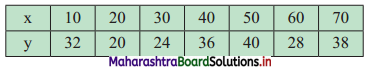Solution: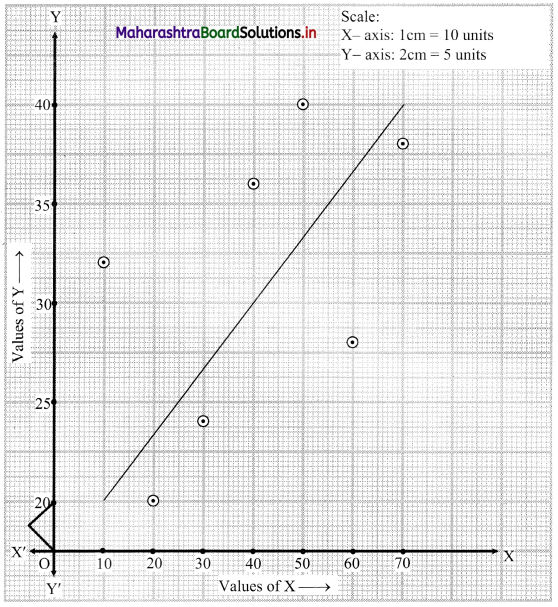Since all the points lie in a band rising from left to right.
Therefore, there is a positive correlation between the values of X and Y respectively.

Question 2.
For the following data of marks of 7 students in Physics (x) and Mathematics (y), draw scatter diagram and state the type of correlation.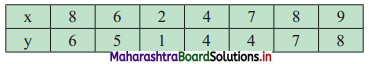Solution:
We take marks in Physics on X-axis and marks in Mathematics on Y-axis and plot the points as below.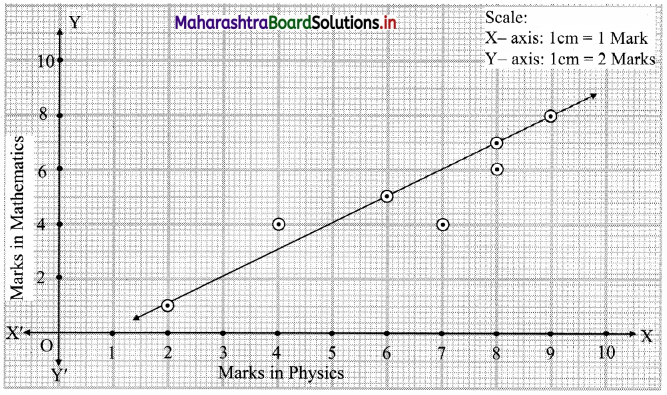We get a band of points rising from left to right. This indicates the positive correlation between marks in Physics and marks in Mathematics.

Question 3.
Draw a scatter diagram for the data given below. Is there any correlation between Aptitude score and Grade points?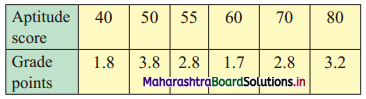Solution: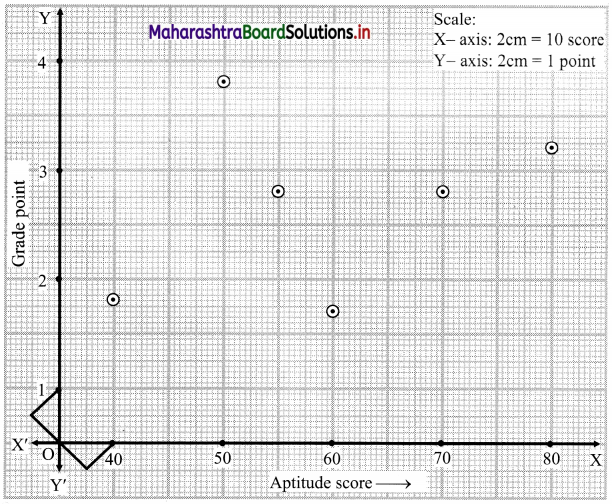The points are completely scattered i.e., no trend is observed.
∴ there is no correlation between Aptitude score (X) and Grade point (Y).

Question 4.
Find correlation coefficient between x andy series for the following data:
n = 15, $$\bar{x}$$ = 25, $$\bar{y}$$ = 18, σx = 3.01, σy = 3.03, $$\sum\left(x_{\mathrm{i}}-\bar{x}\right)\left(y_{\mathrm{i}}-\bar{y}\right)$$ = 122
Solution:
Here, n = 15, $$\bar{x}$$ = 25, $$\bar{y}$$ = 18, σx = 3.01, σy = 3.03, $$\sum\left(x_{\mathrm{i}}-\bar{x}\right)\left(y_{\mathrm{i}}-\bar{y}\right)$$ = 122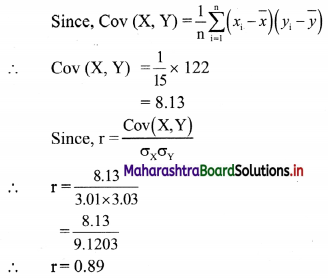Question 5.
The correlation coefficient between two variables x and y are 0.48. The covariance is 36 and the variance of x is 16. Find the standard deviation of y.
Solution:
Given, r = 0.48, Cov(X, Y) = 36
Since $$\sigma_{X}^{2}$$ = 16
∴ σx = 4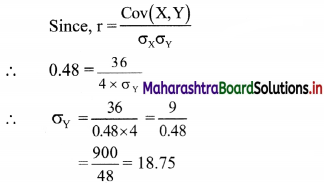∴ the standard deviation of y is 18.75.

Question 6.
In the following data, one of the values of y is missing. Arithmetic means of x and y series are 6 and 8 respectively. (√2 = 1.4142)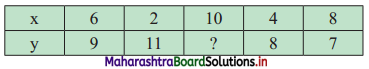(i) Estimate missing observation.
(ii) Calculate correlation coefficient.
Solution:
(i) Let X = xi, Y = yi and missing observation be ‘a’.
Given, $$\bar{x}$$ = 6, $$\bar{y}$$ = 8, n = 5
∴ 8 = $$\frac{35+a}{5}$$
∴ 40 = 35 + a
∴ a = 5
(ii) We construct the following table: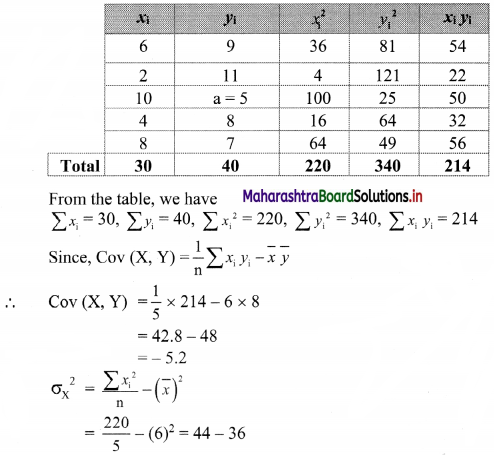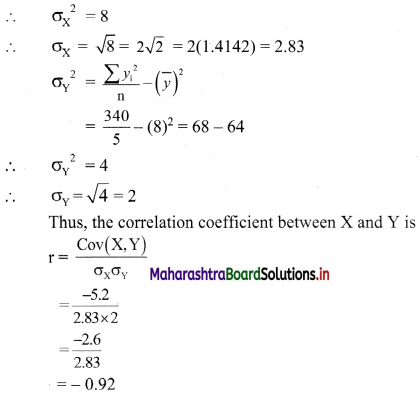Question 7.
Find correlation coefficient from the following data. [Given: √3 = 1.732]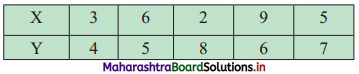Solution: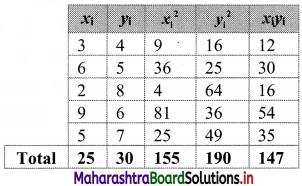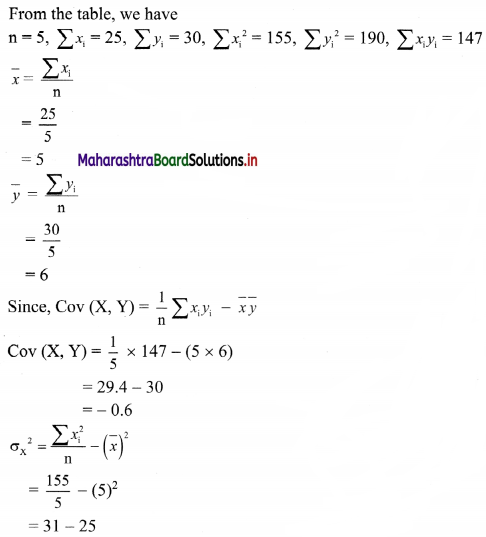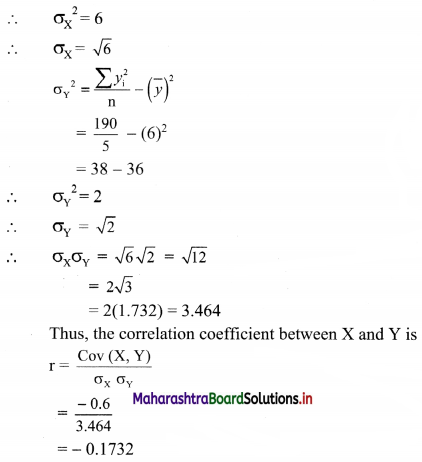Question 8.
The correlation coefficient between x and y is 0.3 and their covariance is 12. The variance of x is 9, find the standard deviation of y.
Solution:
Given, r = 0.3, Cov(X, Y) = 12,
$$\sigma_{X}^{2}$$ = 9
∴ $$\sigma_{\mathrm{X}}$$ = 3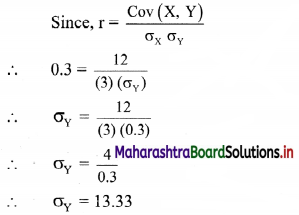∴ the standard deviation of y is 13.33.